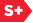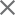### Proceedings Paper

Algebraic and geometric spread in finite frames
Author(s): Emily J. King
Format Member Price Non-Member Price
PDF \$17.00 \$21.00

Paper Abstract

When searching for finite unit norm tight frames (FUNTFs) of M vectors in 𝔽N which yield robust representations, one is concerned with finding frames consisting of frame vectors which are in some sense as spread apart as possible. Algebraic spread and geometric spread are the two most commonly used measures of spread. A frame with optimal algebraic spread is called full spark and is such that any subcollection of N frame vectors is a basis for 𝔽N. A Grassmannian frame is a FUNTF which satisfies the Grassmannian packing problem; that is, the frame vectors are optimally geometrically spread given fixed M and N. A particular example of a Grassmannian frame is an equiangular frame, which is such that the absolute value of all inner products of distinct vectors is equal. The relationship between these two types of optimal spread is complicated. The folk knowledge for many years was that equiangular frames were full spark; however, this is now known not to hold for an infinite class of equiangular frames. The exact relationship between these types of spread will be further explored in this talk, as well as Plücker coordinates and coherence, which are measures of how much a frame misses being optimally algebraically or geometrically spread.

Paper Details

Date Published: 24 August 2015
PDF: 11 pages
Proc. SPIE 9597, Wavelets and Sparsity XVI, 95970B (24 August 2015); doi: 10.1117/12.2188541
Show Author Affiliations
Emily J. King, Univ. of Bremen (Germany)

Published in SPIE Proceedings Vol. 9597:
Wavelets and Sparsity XVI
Manos Papadakis; Vivek K. Goyal; Dimitri Van De Ville, Editor(s)